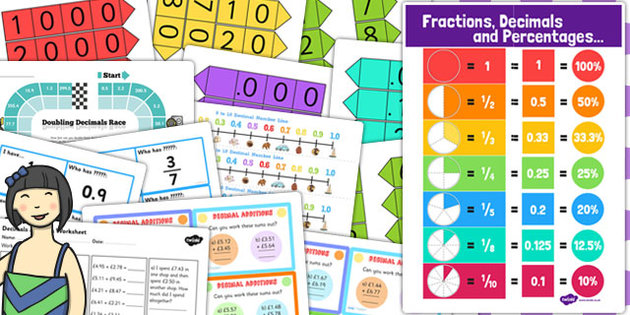Year 7Year 7 Maths includes the following topics:-

Analysing & Displaying Data … tables, charts & diagrams, finding ‘common’ from a set of data or grouped bar chart, finding mode & range, extracting data, interpreting bar charts, using a calculator, using ICT bar graphs & bar-line graphs, collecting data & recording in a frequency table, calculating median, comparing 2 simple distributions, looking at statistics, averages, compound & dual (comparative) bar charts, pie charts, construction of line graphs for time series (frequency polygon), construction & interpretation of scatter graphs, using correlation to describe relations between sets of data, two-way tables.

Calculation & Number Skills … addition & subtraction, strategies, understanding order, number sequences, calculator skills, whole numbers, column procedure, approximation, multiplication, number lines, division, ratio, operations & laws of mathematics, decimals, index notation for squares, cubes & positive integer powers of 10, factor pairs using whole number, HCF & LCM of numbers, square roots, brackets, mental arithmetic, cubed roots & factorising, mental strategies.

Expressions, Functions & Formulae ... outputs of simple functions, linear algebraic expressions, letter symbols, variables, identifying unknowns in a formulae & a function, algebra, complex formula, linear terms, understand roles of letter symbols in formulae & functions, repeated multiplication, expression, common factors.

Graphs, Decimals, Fractions & Measures … interpreting graphs, co-ordinates, conventions & notation, decimals, using symbols, reading scales, calculation, measures & conversions, linear rule, fractions, common denominators, strategies, expressing numbers as fractions, division, multiplication.

Factors, Multiples & Fractions … calculator skills, identifying factors, multiples of numbers, division, rounding numbers, extending written methods, factors, knowing the use & order of operations, primes, fractions, percentages.

Decimals, Measure & Probability … recognising relationship (10’s/100’s), decimal placement, whole numbers, metric units of measurement, decimal notation, recording readings and estimates from scales, length, mass, capacity, rounding numbers & decimals, interpreting scales on a range of measuring instruments, column procedure, consolidating & extending mental methods of calculation, money, probability, division, place value.

Lines, Angles & Shapes … description of angles, compass points, identification of angles and parallel lines, using correct notation, using a protractor, perpendicular lines, estimation, sum, properties & symmetry of 2D shapes, calculation, geometric problems, reasoning, co-ordinates, triangles, polygons, pentagons, quadrilaterals, shape etc.

Fractions, Ratio & Proportion … common denominators, fractions, division, parts of shapes, mental methods of calculation, percentage, decimals, mixed numbers, ratios, metric units, comparison, fractions & linear functions.

Graphs & Sequences … graphs, sequences & functions, co-ordinates, conventions & notation, linear rule & expression, plotting, prediction, comparison, formal algebra, tables, geometric sequence.

Shapes, Perimeter, Area, Volume & Transformations … regular polygons, shapes, using formulae, estimating area, perimeter, line & rotation symmetry, angles, geometric problems, properties, congruence, reflections, rotations, translations, scale factors, nets, conversion, metric & imperial measures, area, formula.

Each student is issued with a workbook at the start of the academic year - open the link below to view a copy of it.